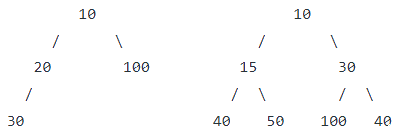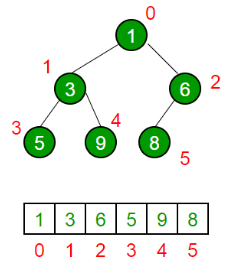# Binary Heap Notes - Class 8

## Class 8: Binary Heap Notes - Class 8

The document Binary Heap Notes - Class 8 is a part of Class 8 category.
All you need of Class 8 at this link: Class 8

Binary Heap
A Binary Heap is a Binary Tree with following properties.

• It’s a complete tree (All levels are completely filled except possibly the last level and the last level has all keys as left as possible). This property of Binary Heap makes them suitable to be stored in an array.
• A Binary Heap is either Min Heap or Max Heap. In a Min Binary Heap, the key at root must be minimum among all keys present in Binary Heap. The same property must be recursively true for all nodes in Binary Tree. Max Binary Heap is similar to MinHeap.

Examples of Min Heap:How is Binary Heap represented?
A Binary Heap is a Complete Binary Tree. A binary heap is typically represented as an array.

• The root element will be at Arr.
• Below table shows indexes of other nodes for the ith node, i.e., Arr[i]:

Arr[(i-1)/2]    Returns the parent node
Arr[(2*i)+1]    Returns the left child node
Arr[(2*i)+2]    Returns the right child node

The traversal method use to achieve Array representation is Level OrderApplications of Heaps:

• Heap Sort: Heap Sort uses Binary Heap to sort an array in O(nLogn) time.
• Priority Queue: Priority queues can be efficiently implemented using Binary Heap because it supports insert(), delete() and extractmax(), decreaseKey() operations in O(logn) time. Binomoial Heap and Fibonacci Heap are variations of Binary Heap. These variations perform union also efficiently.
• Graph Algorithms: The priority queues are especially used in Graph Algorithms like Dijkstra’s Shortest Path and Prim’s Minimum Spanning Tree.
• Many problems can be efficiently solved using Heaps. See following for example.
(i) K’th Largest Element in an array.
(ii) Sort an almost sorted array/
(iii) Merge K Sorted Arrays.

Operations on Min Heap:

• getMini(): It returns the root element of Min Heap. Time Complexity of this operation is O(1).
• extractMin(): Removes the minimum element from MinHeap. Time Complexity of this Operation is O(Logn) as this operation needs to maintain the heap property (by calling heapify()) after removing root.
• decreaseKey(): Decreases value of key. The time complexity of this operation is O(Logn). If the decreases key value of a node is greater than the parent of the node, then we don’t need to do anything. Otherwise, we need to traverse up to fix the violated heap property.
• insert(): Inserting a new key takes O(Logn) time. We add a new key at the end of the tree. IF new key is greater than its parent, then we don’t need to do anything. Otherwise, we need to traverse up to fix the violated heap property.
• delete(): Deleting a key also takes O(Logn) time. We replace the key to be deleted with minum infinite by calling decreaseKey(). After decreaseKey(), the minus infinite value must reach root, so we call extractMin() to remove the key.

Below is the implementation of basic heap operations.

C++

// A C++ program to demonstrate common Binary Heap Operations

#include<iostream>

#include<climits>

using namespace std;

// Prototype of a utility function to swap two integers

void swap(int *x, int *y);

// A class for Min Heap

class MinHeap

{

int *harr; // pointer to array of elements in heap

int capacity; // maximum possible size of min heap

int heap_size; // Current number of elements in min heap

public:

// Constructor

MinHeap(int capacity);

// to heapify a subtree with the root at given index

void MinHeapify(int );

int parent(int i) { return (i-1)/2; }

// to get index of left child of node at index i

int left(int i) { return (2*i + 1); }

// to get index of right child of node at index i

int right(int i) { return (2*i + 2); }

// to extract the root which is the minimum element

int extractMin();

// Decreases key value of key at index i to new_val

void decreaseKey(int i, int new_val);

// Returns the minimum key (key at root) from min heap

int getMin() { return harr; }

// Deletes a key stored at index i

void deleteKey(int i);

// Inserts a new key 'k'

void insertKey(int k);

};

// Constructor: Builds a heap from a given array a[] of given size

MinHeap::MinHeap(int cap)

{

heap_size = 0;

capacity = cap;

harr = new int[cap];

}

// Inserts a new key 'k'

void MinHeap::insertKey(int k)

{

if (heap_size == capacity)

{

cout << "\nOverflow: Could not insertKey\n";

return;

}

// First insert the new key at the end

heap_size++;

int i = heap_size - 1;

harr[i] = k;

// Fix the min heap property if it is violated

while (i != 0 && harr[parent(i)] > harr[i])

{

swap(&harr[i], &harr[parent(i)]);

i = parent(i);

}

}

// Decreases value of key at index 'i' to new_val.  It is assumed that

// new_val is smaller than harr[i].

void MinHeap::decreaseKey(int i, int new_val)

{

harr[i] = new_val;

while (i != 0 && harr[parent(i)] > harr[i])

{

swap(&harr[i], &harr[parent(i)]);

i = parent(i);

}

}

// Method to remove minimum element (or root) from min heap

int MinHeap::extractMin()

{

if (heap_size <= 0)

return INT_MAX;

if (heap_size == 1)

{

heap_size--;

return harr;

}

// Store the minimum value, and remove it from heap

int root = harr;

harr = harr[heap_size-1];

heap_size--;

MinHeapify(0);

return root;

}

// This function deletes key at index i. It first reduced value to minus

// infinite, then calls extractMin()

void MinHeap::deleteKey(int i)

{

decreaseKey(i, INT_MIN);

extractMin();

}

// A recursive method to heapify a subtree with the root at given index

// This method assumes that the subtrees are already heapified

void MinHeap::MinHeapify(int i)

{

int l = left(i);

int r = right(i);

int smallest = i;

if (l < heap_size && harr[l] < harr[i])

smallest = l;

if (r < heap_size && harr[r] < harr[smallest])

smallest = r;

if (smallest != i)

{

swap(&harr[i], &harr[smallest]);

MinHeapify(smallest);

}

}

// A utility function to swap two elements

void swap(int *x, int *y)

{

int temp = *x;

*x = *y;

*y = temp;

}

// Driver program to test above functions

int main()

{

MinHeap h(11);

h.insertKey(3);

h.insertKey(2);

h.deleteKey(1);

h.insertKey(15);

h.insertKey(5);

h.insertKey(4);

h.insertKey(45);

cout << h.extractMin() << " ";

cout << h.getMin() << " ";

h.decreaseKey(2, 1);

cout << h.getMin();

return 0;

}

Python

# A Python program to demonstrate common binary heap operations

# Import the heap functions from python library

from heapq import heappush, heappop, heapify

# heappop - pop and return the smallest element from heap

# heappush - push the value item onto the heap, maintaining

#             heap invarient

# heapify - transform list into heap, in place, in linear time

# A class for Min Heap

class MinHeap:

# Constructor to initialize a heap

def __init__(self):

self.heap = []

def parent(self, i):

return (i-1)/2

# Inserts a new key 'k'

def insertKey(self, k):

heappush(self.heap, k)

# Decrease value of key at index 'i' to new_val

# It is assumed that new_val is smaller than heap[i]

def decreaseKey(self, i, new_val):

self.heap[i]  = new_val

while(i != 0 and self.heap[self.parent(i)] > self.heap[i]):

# Swap heap[i] with heap[parent(i)]

self.heap[i] , self.heap[self.parent(i)] = (

self.heap[self.parent(i)], self.heap[i])

# Method to remove minium element from min heap

def extractMin(self):

return heappop(self.heap)

# This functon deletes key at index i. It first reduces

# value to minus infinite and then calls extractMin()

def deleteKey(self, i):

self.decreaseKey(i, float("-inf"))

self.extractMin()

# Get the minimum element from the heap

def getMin(self):

return self.heap

# Driver pgoratm to test above function

heapObj = MinHeap()

heapObj.insertKey(3)

heapObj.insertKey(2)

heapObj.deleteKey(1)

heapObj.insertKey(15)

heapObj.insertKey(5)

heapObj.insertKey(4)

heapObj.insertKey(45)

print heapObj.extractMin(),

print heapObj.getMin(),

heapObj.decreaseKey(2, 1)

print heapObj.getMin()

# This code is contributed by Nikhil Kumar Singh(nickzuck_007)

C#

// C# program to demonstrate common

// Binary Heap Operations - Min Heap

using System;

// A class for Min Heap

class MinHeap{

// To store array of elements in heap

public int[] heapArray{ get; set; }

// max size of the heap

public int capacity{ get; set; }

// Current number of elements in the heap

public int current_heap_size{ get; set; }

// Constructor

public MinHeap(int n)

{

capacity = n;

heapArray = new int[capacity];

current_heap_size = 0;

}

// Swapping using reference

public static void Swap<T>(ref T lhs, ref T rhs)

{

T temp = lhs;

lhs = rhs;

rhs = temp;

}

// Get the Parent index for the given index

public int Parent(int key)

{

return (key - 1) / 2;

}

// Get the Left Child index for the given index

public int Left(int key)

{

return 2 * key + 1;

}

// Get the Right Child index for the given index

public int Right(int key)

{

return 2 * key + 2;

}

// Inserts a new key

public bool insertKey(int key)

{

if (current_heap_size == capacity)

{

// heap is full

return false;

}

// First insert the new key at the end

int i = current_heap_size;

heapArray[i] = key;

current_heap_size++;

// Fix the min heap property if it is violated

while (i != 0 && heapArray[i] <

heapArray[Parent(i)])

{

Swap(ref heapArray[i],

ref heapArray[Parent(i)]);

i = Parent(i);

}

return true;

}

// Decreases value of given key to new_val.

// It is assumed that new_val is smaller

// than heapArray[key].

public void decreaseKey(int key, int new_val)

{

heapArray[key] = new_val;

while (key != 0 && heapArray[key] <

heapArray[Parent(key)])

{

Swap(ref heapArray[key],

ref heapArray[Parent(key)]);

key = Parent(key);

}

}

// Returns the minimum key (key at

// root) from min heap

public int getMin()

{

return heapArray;

}

// Method to remove minimum element

// (or root) from min heap

public int extractMin()

{

if (current_heap_size <= 0)

{

return int.MaxValue;

}

if (current_heap_size == 1)

{

current_heap_size--;

return heapArray;

}

// Store the minimum value,

// and remove it from heap

int root = heapArray;

heapArray = heapArray[current_heap_size - 1];

current_heap_size--;

MinHeapify(0);

return root;

}

// This function deletes key at the

// given index. It first reduced value

// to minus infinite, then calls extractMin()

public void deleteKey(int key)

{

decreaseKey(key, int.MinValue);

extractMin();

}

// A recursive method to heapify a subtree

// with the root at given index

// This method assumes that the subtrees

public void MinHeapify(int key)

{

int l = Left(key);

int r = Right(key);

int smallest = key;

if (l < current_heap_size &&

heapArray[l] < heapArray[smallest])

{

smallest = l;

}

if (r < current_heap_size &&

heapArray[r] < heapArray[smallest])

{

smallest = r;

}

if (smallest != key)

{

Swap(ref heapArray[key],

ref heapArray[smallest]);

MinHeapify(smallest);

}

}

// Increases value of given key to new_val.

// It is assumed that new_val is greater

// than heapArray[key].

// Heapify from the given key

public void increaseKey(int key, int new_val)

{

heapArray[key] = new_val;

MinHeapify(key);

}

// Changes value on a key

public void changeValueOnAKey(int key, int new_val)

{

if (heapArray[key] == new_val)

{

return;

}

if (heapArray[key] < new_val)

{

increaseKey(key, new_val);

} else

{

decreaseKey(key, new_val);

}

}

}

static class MinHeapTest{

// Driver code

public static void Main(string[] args)

{

MinHeap h = new MinHeap(11);

h.insertKey(3);

h.insertKey(2);

h.deleteKey(1);

h.insertKey(15);

h.insertKey(5);

h.insertKey(4);

h.insertKey(45);

Console.Write(h.extractMin() + " ");

Console.Write(h.getMin() + " ");

h.decreaseKey(2, 1);

Console.Write(h.getMin());

}

}

// This code is contributed by

// Dinesh Clinton Albert(dineshclinton)

Output:

2 4 1

The document Binary Heap Notes - Class 8 is a part of Class 8 category.
All you need of Class 8 at this link: Class 8Use Code STAYHOME200 and get INR 200 additional OFF

Track your progress, build streaks, highlight & save important lessons and more!

,

,

,

,

,

,

,

,

,

,

,

,

,

,

,

,

,

,

,

,

,

;